Empirical ProbabilitiesCustom SearchEMPIRICAL PROBABILITIES Among the most important applications of probability are those situations where we cannot list all possible outcomes. To this point, we have considered problems in which the probabilities could be obtained from situations of equally likely reŁsults. Because some problems are so comŁplicated for analysis, we can only estimate probabilities from experience and obserŁvation. This is empirical probability. In modern industry probability now plays an important role in many activities. Quality control and reliability of a manufactured article have become exŁtremely important considerations in which probability is used. Table 9-1.-Weather Forecast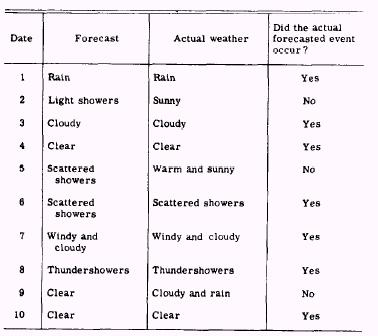Experience has shown that empirical probabilities, if carefully determined on the basis of adequate statistical samples, can be applied to large groups with the result that probability and relative freŁquency are approximately equal. By adeŁquate samples we mean a large enough sample so that accidental runs of "luck," both good and bad, cancel each other. With enough trials, predicted results and actual results agree quite closely. On the other hand, applying a probability ratio to a single individual event is virtually meaningless. We define relative frequency of success as follows: After N trials of an event have been made, of which S trials are successes, the relative frequency of success is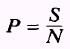For example, table 9-1 shows a small number of weather forecasts from April 1st to April 10th. The actual weather on the dates is also given. Observe that the forecasts on April 1, 3, 4, 6, 7, 8, and 10 were correct. We have observed 10 outcomes. The event of a corŁrect forecast has occurred 7 times. Based on this information we might say that the probability for future forecasts being true is 7/10. This number is the best estimate we can make from the given information. In this case, since we have observed such a small number of outcomes, we would be incorrect to say that the estimate of P is dependable. A great many more cases should be used if we expect to make a good estimate of the probability that a weather forecast will be accurate. A great many factors affect the accuracy of a weather forecast. This example merely indicates something about how successful a particular weather office has been in making weather forecasts. Another example may be drawn from industry. Many thousands of articles of a certain type are manufactured. The comŁpany selects 100 of these articles at random and subjects them to very careful tests. In these tests 98 of the articles are found to meet all measurement requirements and perform satisfactorily. This sugŁgests that 98/100 is a measure of the reliability of the article. One might expect that about 98%Io of all of the articles manufactured by this process will be satisfactory. The probability (measure of chance) that one of these articles will be satisfactory might be said to be 0.98. This second example of empirical probability is different from the first example in one very important respect. In the first example we could list all of the possibilities, and in the second example we could not do so. The selection of a sample and its size is a problem of statistics. Considered from another point of view, statistical probability can be regarded as relative frequency. EXAMPLE: In a dart game, a player hit the bull's eye 3 times out of 25 trials. What is the statistical probability that he will hit the bull's eye on the next throw? SOLUTION: N=25 and S=3 hence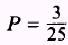EXAMPLE: Using table 9-2, what is the probability that a person 20 years old will live to be 50 years old? SOLUTION: Of 95,148 persons at age 20, 81,090 surŁvived to age 50. HenceEXAMPLE: How many times would a die be exŁpected to land with a 5 or 6 showing in 20 trials? Table 9-2.-Mortality Table (Based on 100,000 Individuals 1 Year of Age)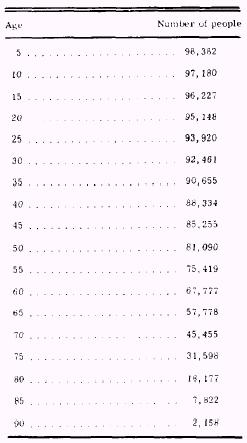SOLUTION: The probability of a 5 or 6 showing is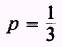The relative frequency is approximately equal to the probabilityTherefore, since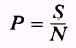where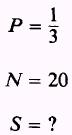then rearranging and substituting, we find that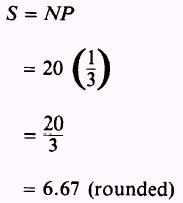This says that the expected number of times a die would land with a 5 or 6 showing in 20 trials is 6.67; that is, on the average a die will land with a 5 or 6 showing 6.67 times per 20 trials. PRACTICE PROBLEMS: l. A construction crew consists of 6 electricians and 38 other workers. How many electricians would you expect to choose if you choose 1 person each day of a workweek for your helper? (Sunday will not be considered part of the workweek.) 2. How many times would a tossed die be expected to turn up a 3 or less in 30 tosses? 3. Using table 9-2, find the probability that a person whose age is 30 will live to age 60. ANSWERS:SUMMARY The following are the major topics covered in this chapter: 1. Probability: Probability is the ratio of the different number of ways a trial can succeed (or fail) to the total number of ways in which it may result. 2. Probabilities of success and failure: If a trial must result in any of n equally likely ways, and if s is the number of sucŁcessful ways and f is the number of failing ways, the probability of success is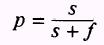and the probability of failure is3. Expectation: Expectation is the average of the values you would get in conducting an experiment or trial exactly the same way many times. 4. Numerical expectation: If the probability of success in one trial is p, and k is the total number of trials, then kp is the expected number of successes in the k trials or5. Mathematical expectation: If, in the event of a successful result, amount a is to be received, and p is the probability of success of that event, then ap is the mathematical expectation or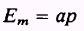6. Independent events: Two or more events are independent if the occurrence or nonoccurrence of one of the events has no effect on the probability of occurrence of any of the others. 7. Dependent events: Two or more events are dependent if the occurrence or nonoccurrence of one of the events affects the probabilities of occurrence of any of the others. 8. Mutually exclusive events: Two or more events are called mutually exclusive if the occurrence of any one of them precludes the occurrence of any of the others. 9. Empirical probability: Empirical probability is an estimated probability from experience and observation. 10. Relative frequency of success: After N trials of an event have been made, of which S trials are successes, the relative freŁquency of success isIntegrated Publishing, Inc. - A (SDVOSB) Service Disabled Veteran Owned Small Business